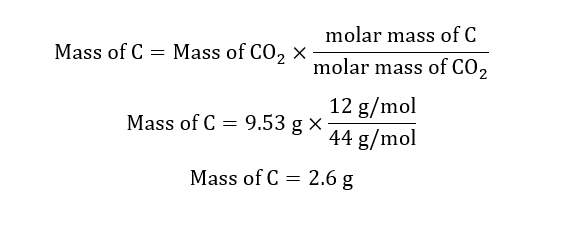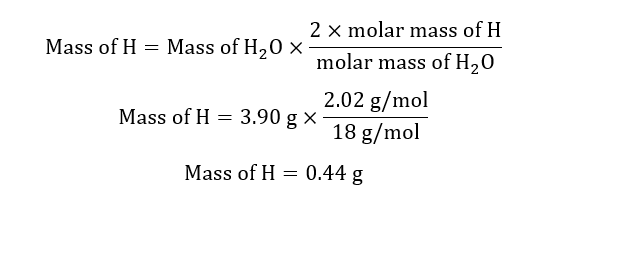# A molecular formula expresses the number of each kind of atom in a molecule. For example, the molecular formula for propene, C3H6C3H6, indicates three carbon atoms and six hydrogen atoms per molecule. This also means that one mole of propene contains three moles of carbon and six moles of hydrogen.An empirical formula expresses the mole ratio of the elements. The empirical formula for propene is CH2CH2, indicating twice as much hydrogen as carbon. When analyzing unknown compounds in a lab, it is often possible to identify the mole ratios, and thus the empirical formula, but not the molecular formula.Notice that the molecular mass of propene, 3(12)+6(1)=42amu3(12)+6(1)=42amu, is a multiple of the empirical formula mass, 1(12)+2(1)=14 amuAn unknown compound contains only carbon, hydrogen, and oxygen (CxHyOzCxHyOz). Combustion of 6.50 gg of this compound produced 9.53 gg of carbon dioxide and 3.90 gg of water.Part AHow many moles of carbon, CC, were in the original sample?Express your answer to three significant figures and include the appropriate units. Part BHow many moles of hydrogen, HH, were in the original sample?Express your answer to three significant figures and include the appropriate units.

Question
50 views

A molecular formula expresses the number of each kind of atom in a molecule. For example, the molecular formula for propene, C3H6C3H6, indicates three carbon atoms and six hydrogen atoms per molecule. This also means that one mole of propene contains three moles of carbon and six moles of hydrogen.An empirical formula expresses the mole ratio of the elements. The empirical formula for propene is CH2CH2, indicating twice as much hydrogen as carbon. When analyzing unknown compounds in a lab, it is often possible to identify the mole ratios, and thus the empirical formula, but not the molecular formula.Notice that the molecular mass of propene, 3(12)+6(1)=42amu3(12)+6(1)=42amu, is a multiple of the empirical formula mass, 1(12)+2(1)=14 amu

An unknown compound contains only carbon, hydrogen, and oxygen (CxHyOzCxHyOz). Combustion of 6.50 gg of this compound produced 9.53 gg of carbon dioxide and 3.90 gg of water.

#### Part A

How many moles of carbon, CC, were in the original sample?
Express your answer to three significant figures and include the appropriate units.

#### Part B

How many moles of hydrogen, HH, were in the original sample?
Express your answer to three significant figures and include the appropriate units.
check_circle

Step 1

Given:

Mass of compound = 6.50 g

Mass of CO2 = 9.53 g.

Mass of H2O = 3.90 g.

Molar mass of CO2 = 44 g/mol.

Molar mass of H2O = 18 g/mol.

Step 2

Calculation for mass of Carbon in CO2:Calculation for mass of Hydrogen in H2O:Step 3

Calculation for mass of O in compound:

Mass of O = mass of compound X – mass of H – ma...

### Want to see the full answer?

See Solution

#### Want to see this answer and more?

Solutions are written by subject experts who are available 24/7. Questions are typically answered within 1 hour.*

See Solution
*Response times may vary by subject and question.
Tagged in

### Chemistry